Latest Banking jobs   »

# Quantitative Aptitude Quiz For RBI Grade B Phase 1 2023 -17th May

Q1. Ratio between efficiency of Arun, Yash and Rana is 6:4:5. All three starts to work together with same efficiency. But Rana, destroys his 60% of work in every evening, due to which they have to work 20 more days then estimated time. Find the estimated days taken by them to complete the work.
(a) 80
(b) 76
(c) 84
(d) 72
(e) 90

Q2. There are 3 pipes A, B and C. A can fill a bucket in 8 minutes. C can fill 18/5 buckets in 18 minutes & pipe B can fill 3/20 buckets in a minute. These pipes are opened in a tank alternatively, 1 minute each starting from A, then C, then B. If tank is filled after 2 hours, then find the volume of tank if the capacity of bucket is 5 liters.
(a) 88 liters
(b) 95 liters
(c) 90 liters
(d) 105 liters
(e) 92 liters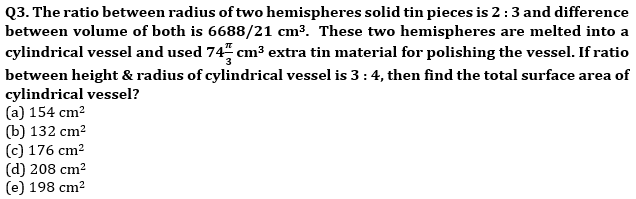Q4. In a box some Munch chocolate, some Dairy milk chocolate and Kit Kat chocolate. The probability of selecting one Munch chocolate out of box is 3/10 and the probability of selecting one Kit Kat chocolate out of box is 2/5. The number of Dairy milk chocolate in box is 6. Find probability of selecting three chocolate from the box such that at most two chocolate are same?
(a) 64/85
(b) 64/95
(c) 42/85
(d) 87/95
(e) 67/85

Q5. There are three vessels A, B & C having Milk to water ratio as 3 : 5, 1 : 3 and 7 : y. After adding mixture of vessel B to A, the milk to water ratio becomes 1 : 2 in A . Now 60% of mixture is taken out from vessel A and mixture of vessel C is mixed with remaining mixture of vessel A which results in final Milk to Water ratio of 3 : 4. Find the value of y given that volume of B is 20 ltrs and volume of C is (7 + y) ltrs.
(a) 1
(b) 4
(c) 5
(d) 3
(e) 2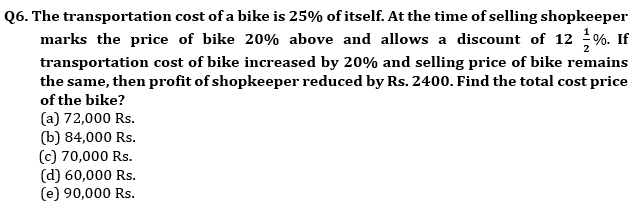Q7.A man invested an amount into three schemes P, Q and R in the ratio of 5 : 6 : 9 at the rates of 20%, 15% and 10% respectively. Schemes P and R offered compound interest annually, while scheme Q offered simple interest annually. Total interest received by man from scheme P and Q together is Rs. 1899 more than total interest received from scheme R after two years. If man would invest total amount on C.I at the rate of 15% p.a., then find the total interest received by the man?
(a) 5800 Rs.
(b) 5805 Rs.
(c) 5580 Rs.
(d) 5590 Rs.
(e) 5900 Rs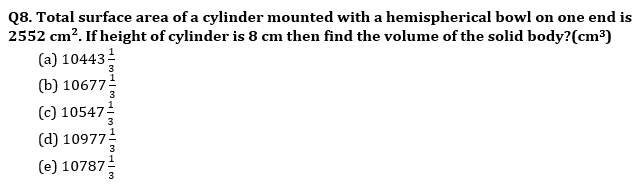Q9. Train A running at the speed of 108 km/hr crosses a man, who running in the opposite direction at the speed of 12 km/hr in 7.2 sec. If speed of train A increased by 25% and it takes 48 seconds to cross another train B, which running at the speed of 90 km/hr in same direction. Find the length of train B?
(a) 280 meters
(b) 360 meters
(c) 180 meters
(d) 160 meters
(e) 220 meters
Q10. There are two buckets, which contains some fruits. First bucket contains three mangos and six oranges, second bucket contains seven mangos and two oranges. One bucket is selected at random and one fruit is drawn from it. Find the probability of selected fruit is not mango?
(a) 2/9
(b) 5/9
(c) 1/9
(d) 4/9
(e) 7/9

Solutions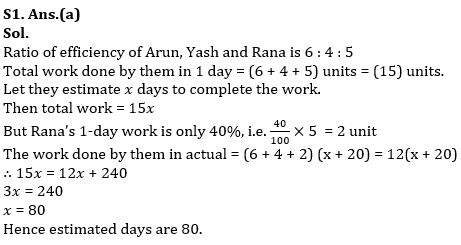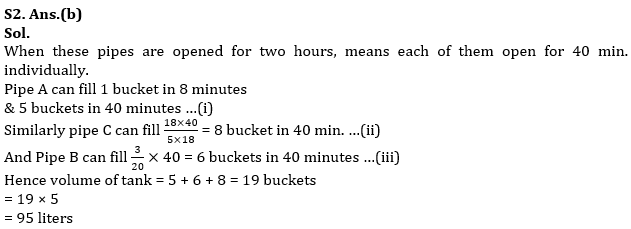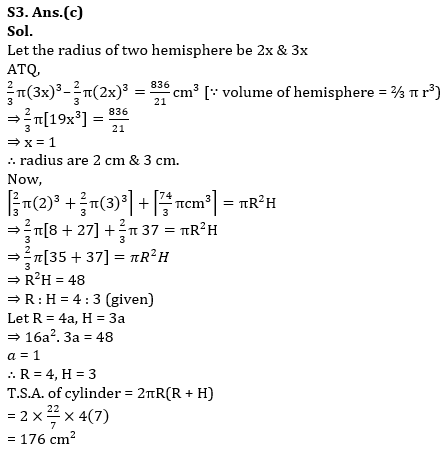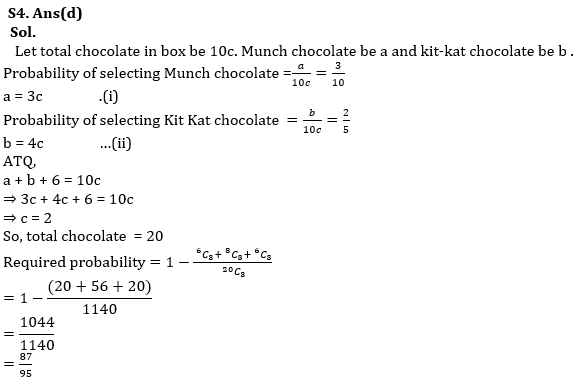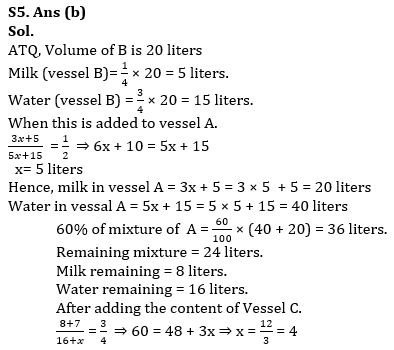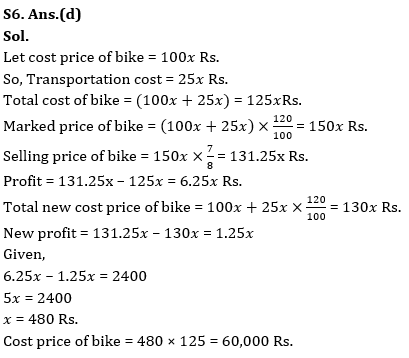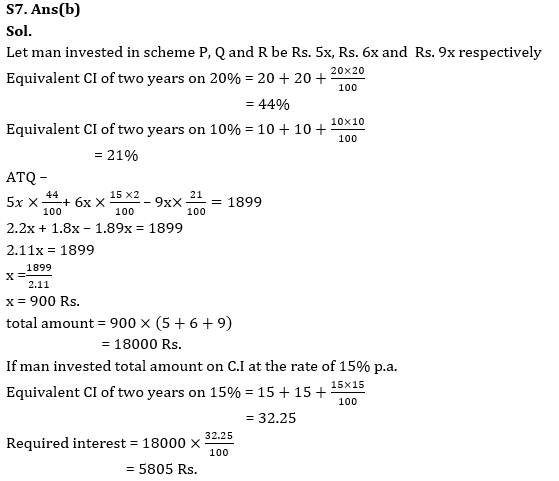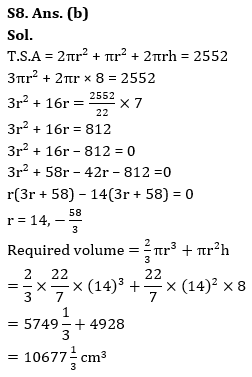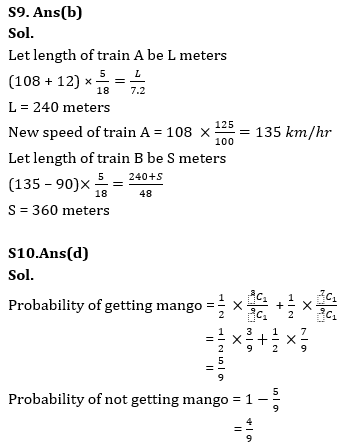## FAQs

### How many sections are there in the RBI Grade B Phase 1 Exam?

There are 4 sections in the RBI Grade B Phase 1 Exam i.e. English Language, General Awareness, Quantitative Aptitude & Reasoning.

#### Congratulations!Union Budget 2023-24: Free PDF